# Nuclear chemistry Questions and Answers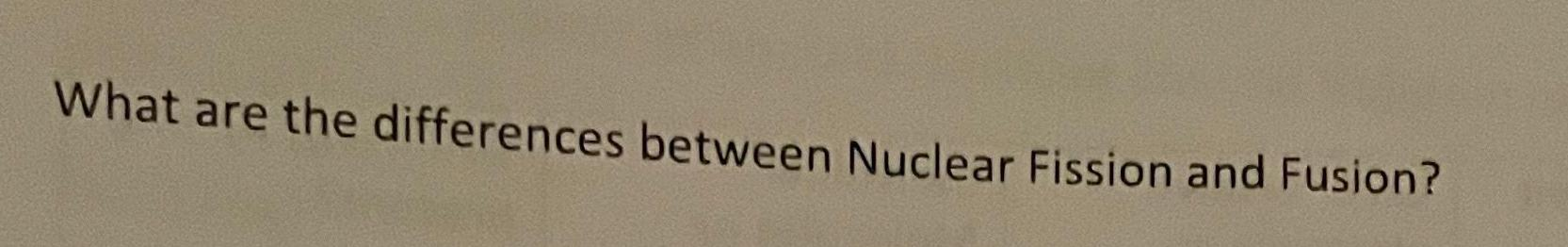Physical Chemistry
Nuclear chemistry
What are the differences between Nuclear Fission and Fusion?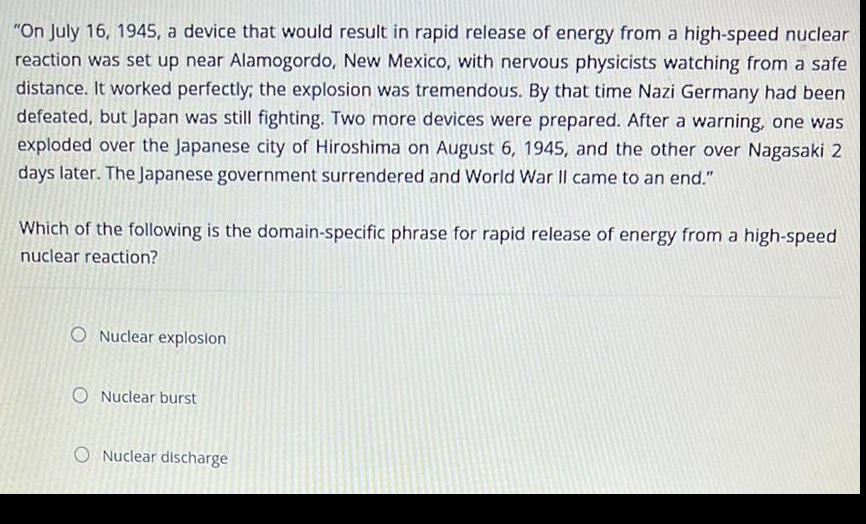Physical Chemistry
Nuclear chemistry
"On July 16, 1945, a device that would result in rapid release of energy from a high-speed nuclear reaction was set up near Alamogordo, New Mexico, with nervous physicists watching from a safe distance. It worked perfectly; the explosion was tremendous. By that time Nazi Germany had been defeated, but Japan was still fighting. Two more devices were prepared. After a warning, one was exploded over the Japanese city of Hiroshima on August 6, 1945, and the other over Nagasaki 2 days later. The Japanese government surrendered and World War II came to an end." Which of the following is the domain-specific phrase for rapid release of energy from a high-speed nuclear reaction? Nuclear explosion Nuclear burst Nuclear discharge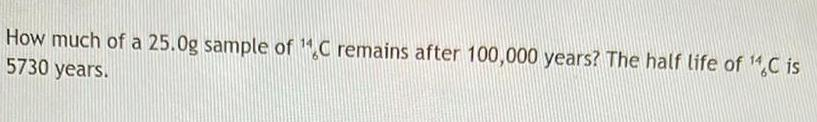Physical Chemistry
Nuclear chemistry
How much of a 25.0g sample of C remains after 100,000 years? The half life of 14C6 is 5730 years.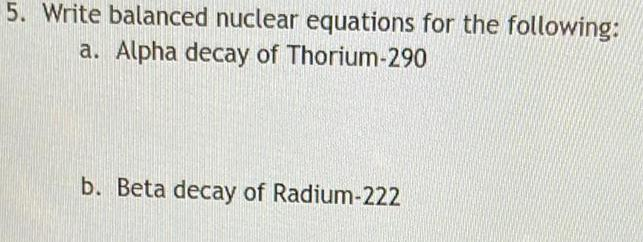Physical Chemistry
Nuclear chemistry
Write balanced nuclear equations for the following: a. Alpha decay of Thorium-290 b. Beta decay of Radium-222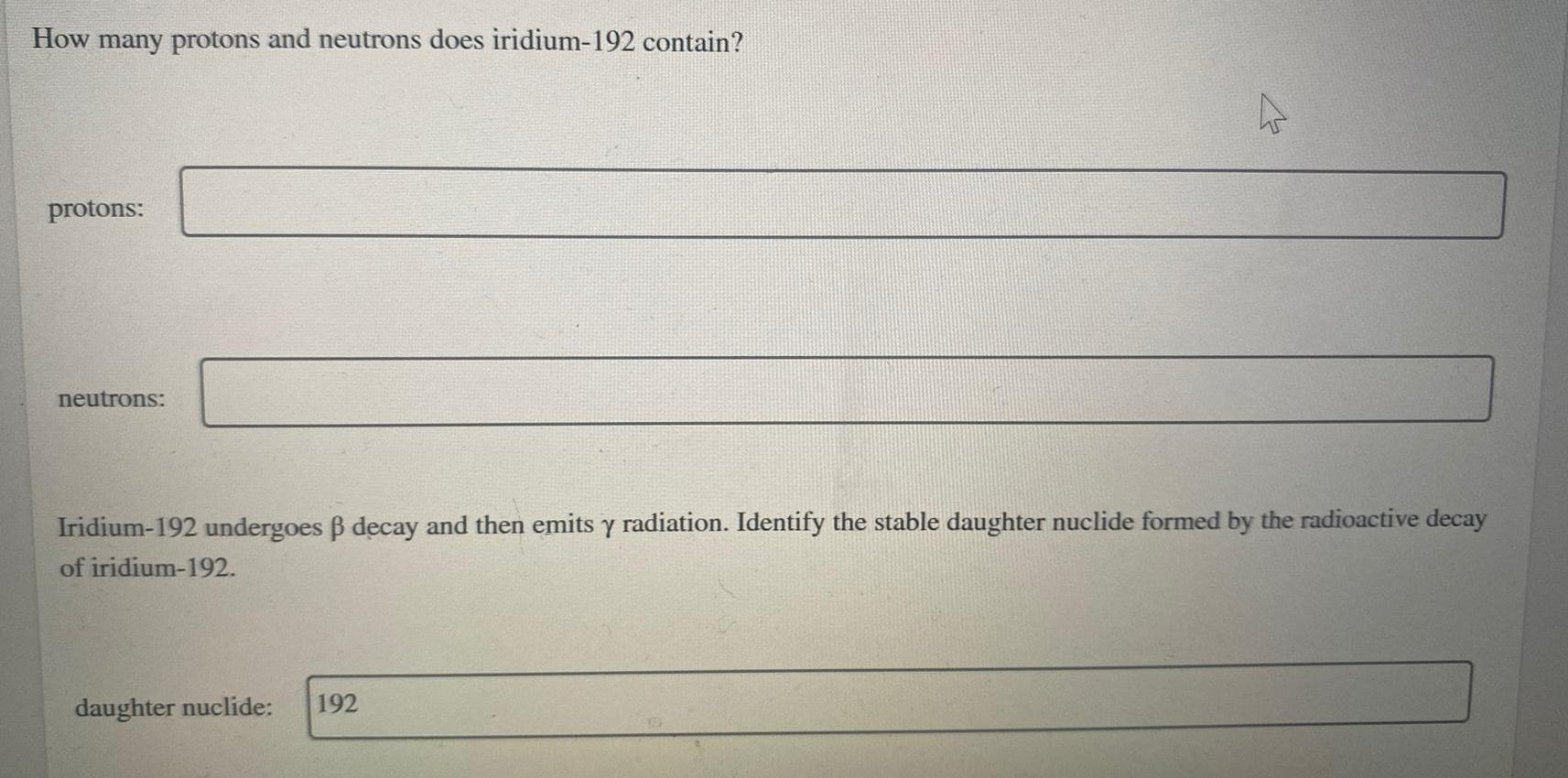Physical Chemistry
Nuclear chemistry
How many protons and neutrons does iridium-192 contain? protons: neutrons: Iridium-192 undergoes ß decay and then emits y radiation. Identify the stable daughter nuclide formed by the radioactive decay of iridium-192. daughter nuclide: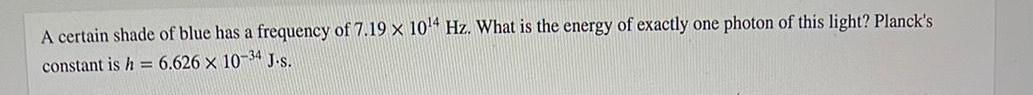Physical Chemistry
Nuclear chemistry
A certain shade of blue has a frequency of 7.19 x 10^14 Hz. What is the energy of exactly one photon of this light? Planck's constant is h = 6.626 x 10-34 J.s.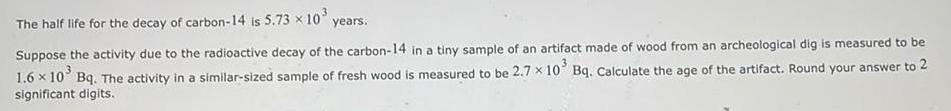Physical Chemistry
Nuclear chemistry
The half life for the decay of carbon-14 is 5.73 × 10³ years. Suppose the activity due to the radioactive decay of the carbon-14 in a tiny sample of an artifact made of wood from an archeological dig is measured to be 1.6 × 10³ Bq. The activity in a similar-sized sample of fresh wood is measured to be 2.7 x 10³ Bq. Calculate the age of the artifact. Round your answer to 2 significant digits.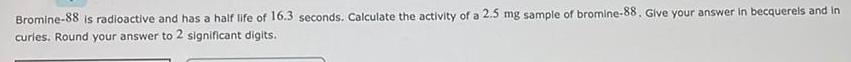Physical Chemistry
Nuclear chemistry
Bromine-88 is radioactive and has a half life of 16.3 seconds. Calculate the activity of a 2.5 mg sample of bromine-88. Give your answer in becquerels and in curies. Round your answer to 2 significant digits.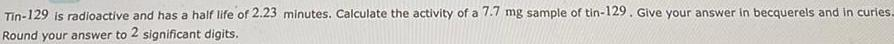Physical Chemistry
Nuclear chemistry
Tin-129 is radioactive and has a half life of 2.23 minutes. Calculate the activity of a 7.7 mg sample of tin-129. Give your answer in becquerels and in curies. Round your answer to 2 significant digits.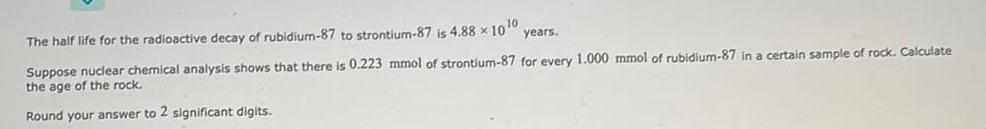Physical Chemistry
Nuclear chemistry
The half life for the radioactive decay of rubidium-87 to strontium-87 is 4.88 × 10¹0 years. Suppose nuclear chemical analysis shows that there is 0.223 mmol of strontium-87 for every 1.000 mmol of rubidium-87 in a certain sample of rock. Calculate the age of the rock. Round your answer to 2 significant digits.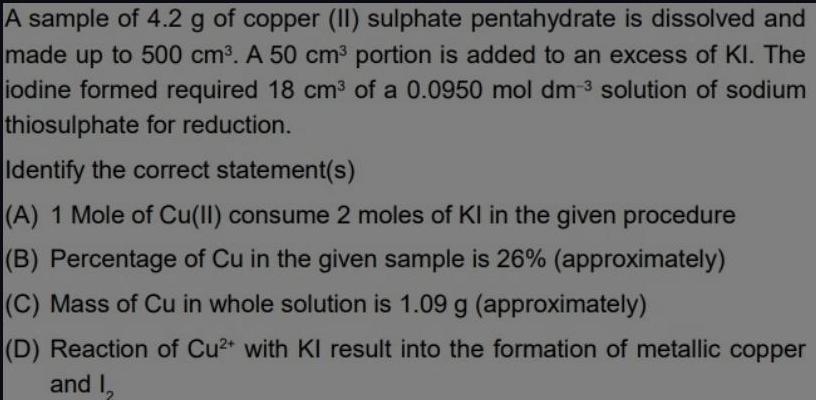Physical Chemistry
Nuclear chemistry
A sample of 4.2 g of copper (II) sulphate pentahydrate is dissolved and made up to 500 cm³. A 50 cm³ portion is added to an excess of Kl. The iodine formed required 18 cm³ of a 0.0950 mol dm-3 solution of sodium thiosulphate for reduction. Identify the correct statement(s) (A) 1 Mole of Cu(II) consume 2 moles of Kl in the given procedure (B) Percentage of Cu in the given sample is 26% (approximately) (C) Mass of Cu in whole solution is 1.09 g (approximately) (D) Reaction of Cu²+ with Kl result into the formation of metallic copper and I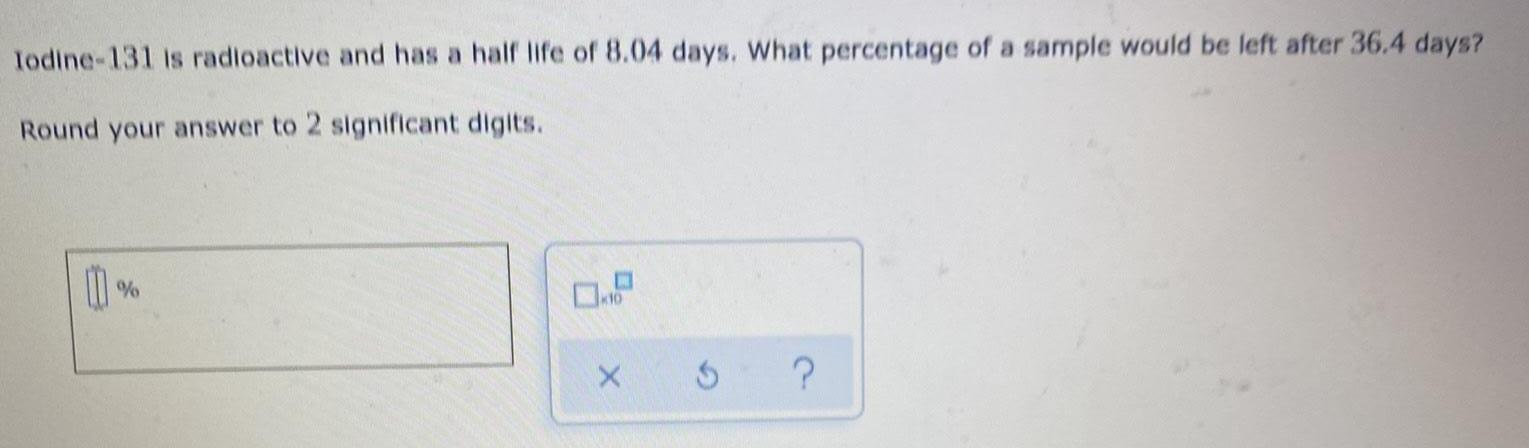Physical Chemistry
Nuclear chemistry
Iodine-131 is radioactive and has a half life of 8.04 days. What percentage of a sample would be left after 36.4 days? Round your answer to 2 significant digits. 00 % ×10 x 3 ?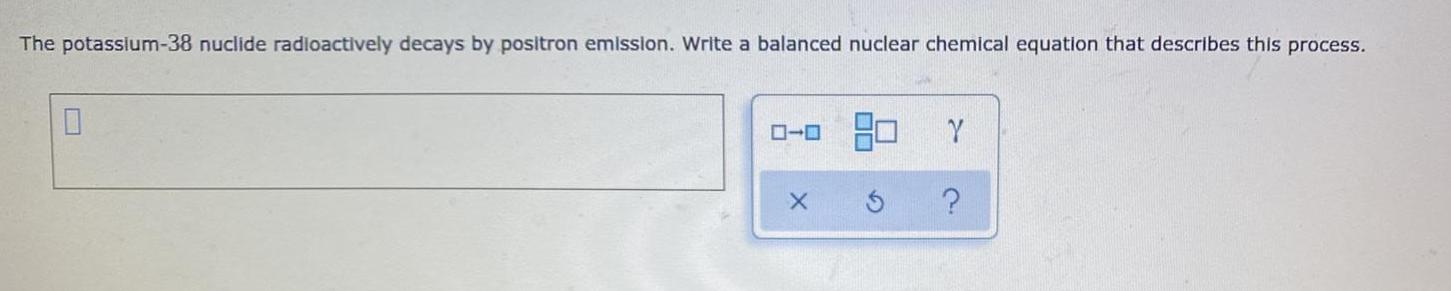Physical Chemistry
Nuclear chemistry
The potassium-38 nuclide radioactively decays by positron emission. Write a balanced nuclear chemical equation that describes this process. X Ś Y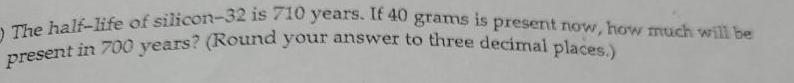Physical Chemistry
Nuclear chemistry
The half-life of silicon-32 is 710 years. If 40 grams is present now, how much will be present in 700 years? (Round your answer to three decimal places.)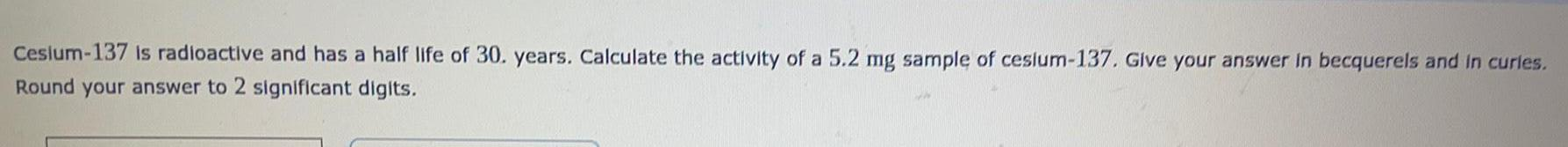Physical Chemistry
Nuclear chemistry
Cesium-137 is radioactive and has a half life of 30. years. Calculate the activity of a 5.2 mg sample of cesium-137. Give your answer in becquerels and in curies. Round your answer to 2 significant digits.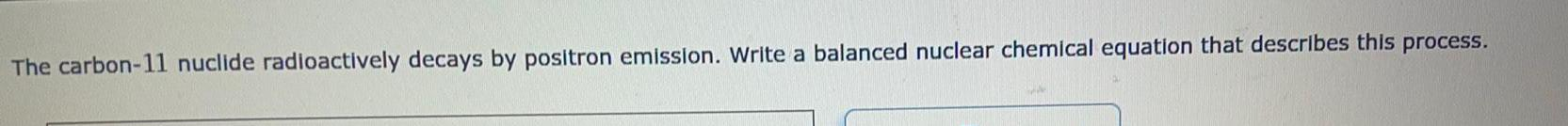Physical Chemistry
Nuclear chemistry
The carbon-11 nuclide radioactively decays by positron emission. Write a balanced nuclear chemical equation that describes this process.